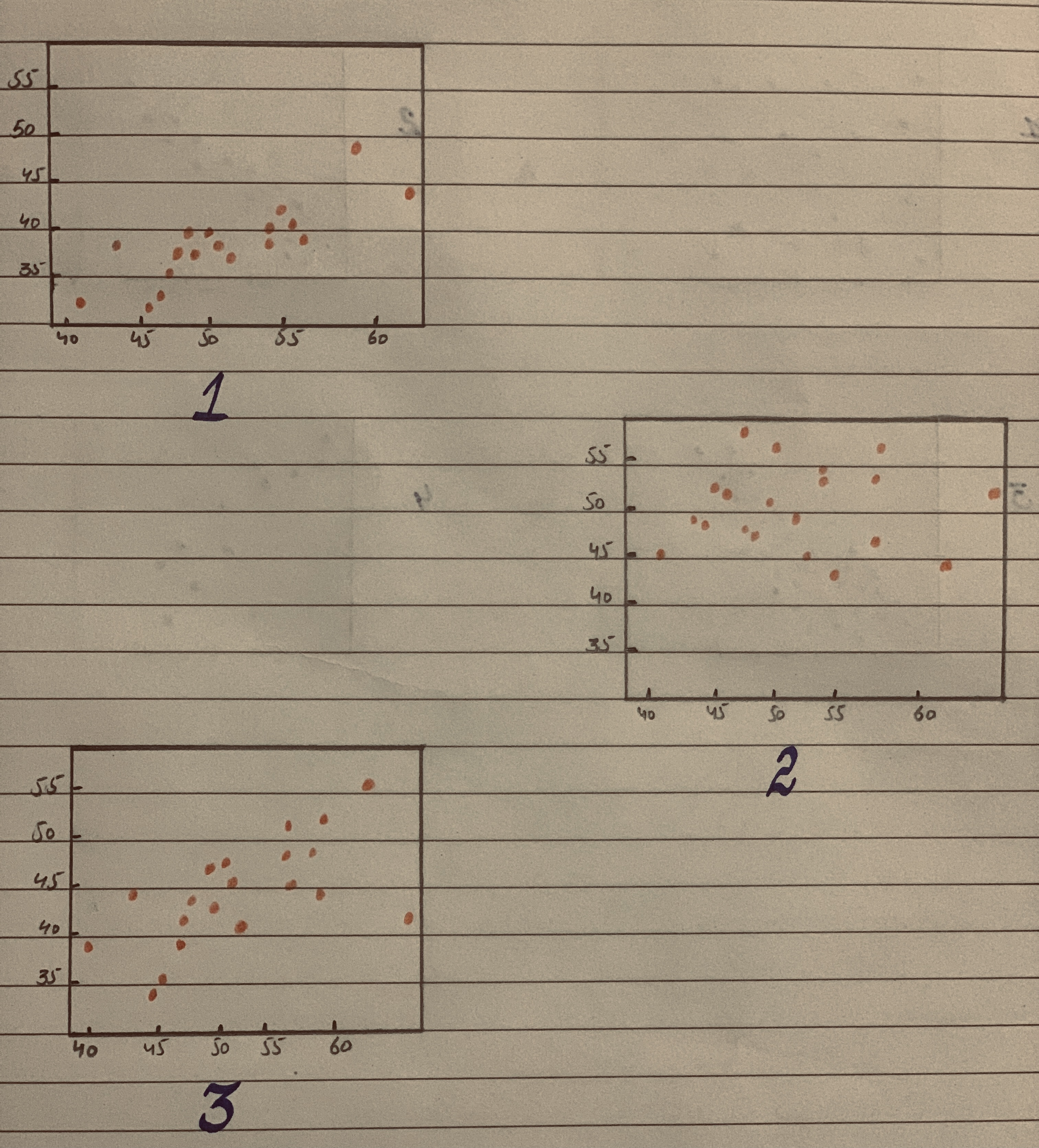# The scatterplots below display three bivariate data sets. The correlation coefficients for these data sets are 0.03, 0.68, and 0.89.beljuA 2020-12-07 Answered

The scatterplots below display three bivariate data sets. The correlation coefficients for these data sets are 0.03, 0.68, and 0.89. Which scatter plot corresponds to the data set with r = .03?You can still ask an expert for help

• Questions are typically answered in as fast as 30 minutes

Solve your problem for the price of one coffee

• Math expert for every subject
• Pay only if we can solve itSally Cresswell
Correlation:
The value of correlation coefficient tells about the strength of the association between two variables. The range of correlation coefficient value is –1 to +1. The value of 0 indicates that there is no correlation between two variables, the value of +1 indicates that there is perfect positive relationship between two variables, the value of –1 indicates that there is perfect negative relationship two variables. higher the correlation coefficient value, higher the correlation between the two variables.
Scatterplot with r=0.03:
In scatterplot 2, the data are more scattered when compared to other two scatterplots. In other words, there is no pattern observed. Whereas, scatterplot 3 is somewhat linear and scatterplot 1 is approximately linear.
Thus, the scatterplot 2 is expected to have the least correlation coefficient value and the scatterplot 1 is expected to have the highest correlation coefficient value among the given values. The correct answer is the scatterplot corresponds to the data set with r=0.03 is scatterplot 2.